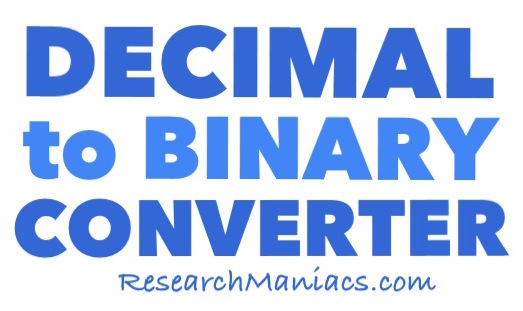How to Convert 30 Decimal to Binary

 Here are step-by-step instructions on how to convert the decimal number 30 to a binary number. Step A) Determine if 30 is an even or odd number. If it is even, you assign a 0 and if it is odd you assign a 1. Step B) Deduct the 1 or 0 in step A from 30 and then divide it by 2. Then, you see if that number is even or odd and then assign a 0 or 1 like step A. Step C) Repeat step B with the new number until the number you get is 1. Step D) Put all the 0s and 1s you assigned in reverse order to get the answer. To illustrate better, we detailed below how we converted 30 decimal to binary using the steps above. 30 → 0(30 - 0)/2 = 15 → 1(15 - 1)/2 = 7 → 1(7 - 1)/2 = 3 → 1(3 - 1)/2 = 1 → 1 Then you put the binary number above in reverse order and you get the answer: 11110Convert Decimal to Binary Enter another decimal below to convert it to binary.

 How to Convert 31 Decimal to Binary On this page we showed you how to convert 30 decimal to binary. Use this knowledge to see if you can convert the next decimal number on our list to binary!